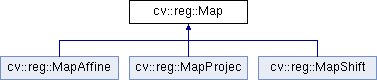OpenCV  4.1.0 Open Source Computer Vision
cv::reg::Map Class Referenceabstract

Base class for modelling a Map between two images. More...

#include <opencv2/reg/map.hpp>

Inheritance diagram for cv::reg::Map:## Public Member Functions

virtual ~Map ()

virtual void compose (cv::Ptr< Map > map)=0

virtual cv::Ptr< MapinverseMap () const =0

virtual void inverseWarp (InputArray img1, OutputArray img2) const =0

virtual void scale (double factor)=0

virtual void warp (InputArray img1, OutputArray img2) const

## Detailed Description

Base class for modelling a Map between two images.

The class is only used to define the common interface for any possible map.

## § ~Map()

 virtual cv::reg::Map::~Map ( )
virtual

Virtual destructor

## § compose()

 virtual void cv::reg::Map::compose ( cv::Ptr< Map > map )
pure virtual
Python:
None=cv.reg_Map.compose(map)

Changes the map composing the current transformation with the one provided in the call. The order is first the current transformation, then the input argument.

Parameters
 [in] map Transformation to compose with.

Implemented in cv::reg::MapShift, cv::reg::MapAffine, and cv::reg::MapProjec.

## § inverseMap()

 virtual cv::Ptr cv::reg::Map::inverseMap ( ) const
pure virtual
Python:
retval=cv.reg_Map.inverseMap()

Calculates the inverse map

Returns
Inverse map

Implemented in cv::reg::MapShift, cv::reg::MapAffine, and cv::reg::MapProjec.

## § inverseWarp()

 virtual void cv::reg::Map::inverseWarp ( InputArray img1, OutputArray img2 ) const
pure virtual
Python:
img2=cv.reg_Map.inverseWarp(img1[, img2])

Warps image to a new coordinate frame. The calculation is img2(x)=img1(T(x)), so in fact this is the inverse warping as we are taking the value of img1 with the forward transformation of the points.

Parameters
 [in] img1 Original image [out] img2 Warped image

Implemented in cv::reg::MapShift, cv::reg::MapAffine, and cv::reg::MapProjec.

## § scale()

 virtual void cv::reg::Map::scale ( double factor )
pure virtual
Python:
None=cv.reg_Map.scale(factor)

Scales the map by a given factor as if the coordinates system is expanded/compressed by that factor.

Parameters
 [in] factor Expansion if bigger than one, compression if smaller than one

Implemented in cv::reg::MapShift, cv::reg::MapAffine, and cv::reg::MapProjec.

## § warp()

 virtual void cv::reg::Map::warp ( InputArray img1, OutputArray img2 ) const
virtual
Python:
img2=cv.reg_Map.warp(img1[, img2])

Warps image to a new coordinate frame. The calculation is img2(x)=img1(T^{-1}(x)), as we have to apply the inverse transformation to the points to move them to were the values of img2 are.

Parameters
 [in] img1 Original image [out] img2 Warped image

The documentation for this class was generated from the following file: